MORE IN Engineering Mechanics (EM)
SPPU First Year Engineering (Semester 2)
Engineering Mechanics (EM)
April 2013
Total marks: --
Total time: --
INSTRUCTIONS
(1) Assume appropriate data and state your reasons
(2) Marks are given to the right of every question
(3) Draw neat diagrams wherever necessary

Answer any one question from Q1 & Q2
1 (a) The resultant of two forces P and Q is 1200 N vertical. Determine the force Q and the corresponding angle ? for the system of forces as shown in Fig. 1a.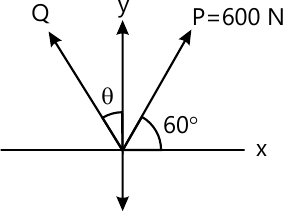4 M
1 (b) The 4.5 × 106 kg tanker is pulled with constant acceleration of 0.001 m/s2 using cable that makes an angle of 15° with the horizontal as shown in in Fig. 1 b. Determine the force in the cable using Newton's second law of motion.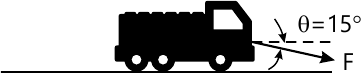4 M
1 (c) During a race the dirt bike was observed to leap up off the small hill at A at an angle of 60° with the horizontal as shown in Fig. 1c. If the point of landing is 6 m away, determine the approximate speed at which the bike was travelling just before it left the ground.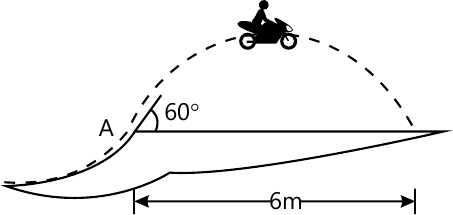4 M
1 (d) A woman having a mass of 70 kg stands in an elevator which has a downward acceleration of 4 m/s2 starting from rest. Determine the work done by her weight and the normal force which the floor exerts on her when the elevator descends 6 m.
4 M

2 (a) Determine the y coordinate of centroid of the shaded area as shown in Fig. 2a.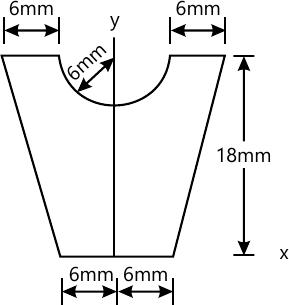4 M
2 (b) A girl having mass of 25kg sits at the edge of the merry go-round so her centre of mass G is at a distance of 1.5 m from the centre of rotation as shown in Fig. 2b. Neglecting tangential component of acceleration, determine the maximum speed which she can have before she begins to slip off the merry go-round. The coefficient of static friction is ?s=0.3 Use Newton's second law of motion.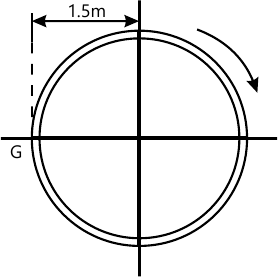4 M
2 (c) A baseball is thrown downward from a 15 m tower with an initial speed of 5 m/s. determine the speed at which it hits the ground and the time of travel.
4 M
2 (d) A ball has a mass of 30 kg and is thrown upward with a speed of 15 m/s. Determine the time to attain maximum height using impulse momentum principle. Also find the maximum height.
4 M

Answer any one question from Q3 & Q4
3 (a) The motor at B winds up the cord attached to the 65 N crate with a constant speed as shown in Fig. 3a. Determine the force in cord CD supporting the pulley and the angle ? for equilibrium. Neglet the size of pulley at C.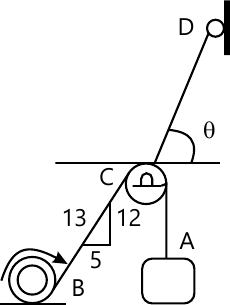6 M
3 (b) The boom supports the two vertical loads P1=800 N and P2=350 N as shown in Fig. 3b. Determine the tension in cable BC and component of reaction at A.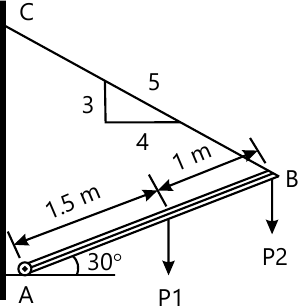6 M
3 (c) A concrete foundation mat in the shape of regular hexagon with 3 m side support column loads as shown inFig. 3c. Determine the magnitude of the additional loads P1 and P2 that must be applied at B and F if resultant of all six loads is to pass through the centre of the mat.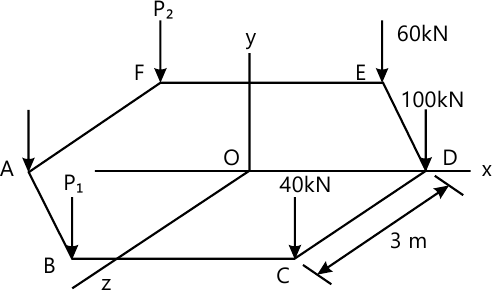5 M

4 (a) The rope BC will fail when the tension becomes 50 kN as shown In Fig. 4A. Determine the greatest load P that can be applied to the beam At B and reaction at A for equilibrium.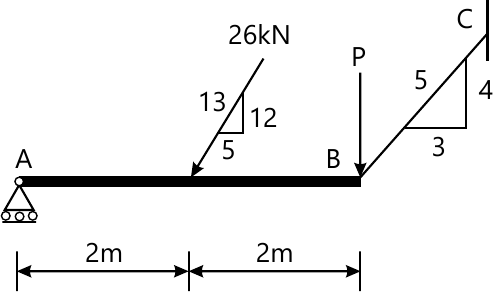6 M
4 (b) The three cables are used to support the 800 N lamp as shown in Fig. 4b. Determine the force developed in each cable for equilibrium.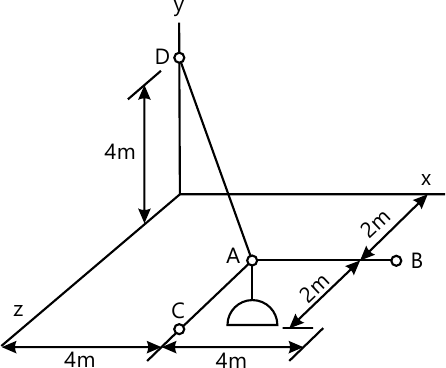6 M
4 (c) State and explain active forces, reactive forces and free body diagram with suitable example.
5 M

Answer any one question from Q5 & Q6
5 (a) Determine the magnitude and nature of forces in the members BC, HC and HG of the truss loaded and supported as shown in Fig. 5a.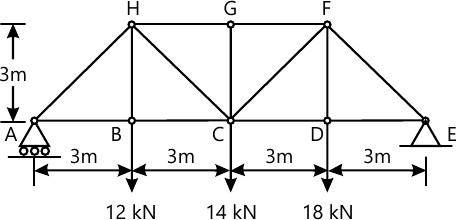6 M
5 (b) The 15 m ladder has a uniform weight of 80 N and rest against the smooth wall at B as shown in Fig. 5b. If the coefficient of static friction ?s=0.4, determine if the ladder will slip?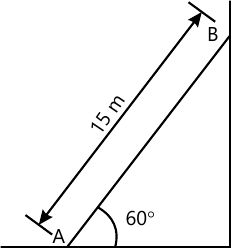6 M
5 (c) Define angle of repose, angle of friction, coefficient of friction and cone of friction with sketches.
5 M

6 (a) Determine the forces in each member of the truss and state if the members are in tension or compression. Refer Fig. 6a.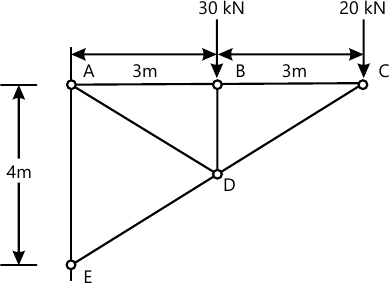6 M
6 (b) Two loads are suspended as shown in Fig. 6b from cable ABCD. Knowing that dc=0.75 m and db=1.125 m, determine the component of reaction at A maximum tension in the cable.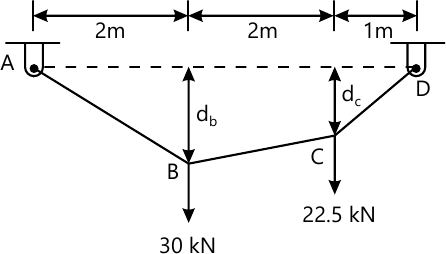6 M
6 (c) A 400 N block is resting on a rough horizontal surface as shown in Fig. 6c for which the coefficient of friction is 0.4. Determine the force P required to cause motion if applied to the block horizontally. What minimum force is required to start motion?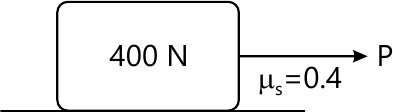5 M

More question papers from Engineering Mechanics (EM)